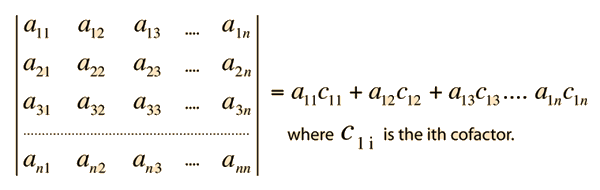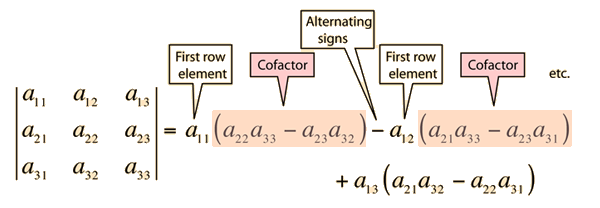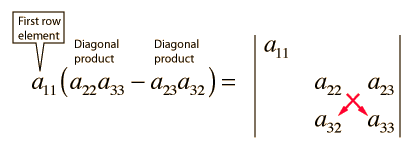# Determinants

A determinant is a square array of elements with a prescribed evaluation rule which is depicted as followsThe evaluation rule can be illustrated by a determinant of order three.

Determinants are useful in the evaluation of vector products and vector operations like the curl.
Index

Vector calculus

 HyperPhysics****HyperMath*****Linear Algebra R Nave
Go Back

# Determinant Evaluation Example

For a determinant of order three the evaluation rule isTake the elements of the top row and multiply them times the determinant of their cofactors. The cofactor is the array left when the row and column of the given top row element is eliminated. The evaluation of the determinant of the cofactor follows the same pattern until the cofactor has dimension two. At that point, it's value is the difference of the diagonal products.Index

Vector calculus

 HyperPhysics****HyperMath*****Linear Algebra R Nave
Go Back